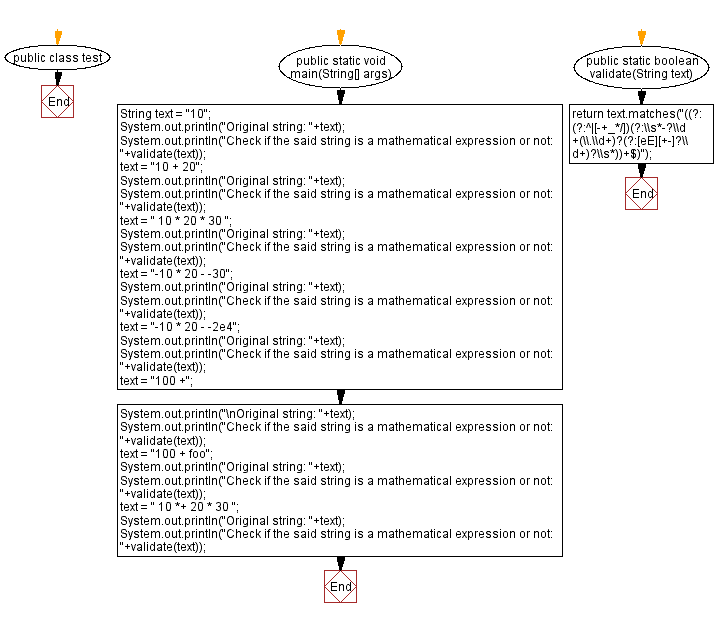﻿ Java: Check if a given string is a mathematical expression# Java programming Exercises: Check if a given string is a mathematical expression

## Java Regular Expression: Exercise-29 with Solution

Write a Java program to check if a given string is a Mathematical Expression or not.

Sample Solution:

Java Code:

``````public class test {
public static void main(String[] args) {
String text = "10";
System.out.println("Original string: "+text);
System.out.println("Check if the said string is a mathematical expression or not: "+validate(text));
text = "10 + 20";
System.out.println("Original string: "+text);
System.out.println("Check if the said string is a mathematical expression or not: "+validate(text));
text = " 10 * 20 * 30 ";
System.out.println("Original string: "+text);
System.out.println("Check if the said string is a mathematical expression or not: "+validate(text));
text = "-10 * 20 - -30";
System.out.println("Original string: "+text);
System.out.println("Check if the said string is a mathematical expression or not: "+validate(text));
text = "-10 * 20 - -2e4";
System.out.println("Original string: "+text);
System.out.println("Check if the said string is a mathematical expression or not: "+validate(text));
text = "100 +";
System.out.println("\nOriginal string: "+text);
System.out.println("Check if the said string is a mathematical expression or not: "+validate(text));
text = "100 + foo";
System.out.println("Original string: "+text);
System.out.println("Check if the said string is a mathematical expression or not: "+validate(text));
text = " 10 *+ 20 * 30 ";
System.out.println("Original string: "+text);
System.out.println("Check if the said string is a mathematical expression or not: "+validate(text));
}

public static boolean validate(String text) {
return text.matches("((?:(?:^|[-+_*/])(?:\\s*-?\\d+(\\.\\d+)?(?:[eE][+-]?\\d+)?\\s*))+\$)");
}
}
```
```

Sample Output:

```Original string: 10
Check if the said string is a mathematical expression or not: true
Original string: 10 + 20
Check if the said string is a mathematical expression or not: true
Original string:  10 * 20 * 30
Check if the said string is a mathematical expression or not: true
Original string: -10 * 20 - -30
Check if the said string is a mathematical expression or not: true
Original string: -10 * 20 - -2e4
Check if the said string is a mathematical expression or not: true

Original string: 100 +
Check if the said string is a mathematical expression or not: false
Original string: 100 + foo
Check if the said string is a mathematical expression or not: false
Original string:  10 *+ 20 * 30
Check if the said string is a mathematical expression or not: false
```

Flowchart :Java Code Editor:

Next: Insert a dash between an upper case letter and a lower case letter in a given string.

What is the difficulty level of this exercise?

Test your Programming skills with w3resource's quiz.

﻿

## Java: Tips of the Day

Split Java String by New Line

```String lines[] = string.split("\\r?\\n");
```

There's only really two newlines (UNIX and Windows) that you need to worry about.

Ref: https://bit.ly/3dHk7ip